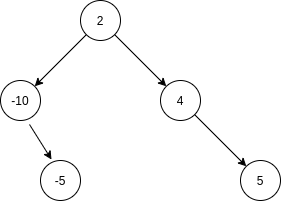New update is available. Click here to update.

# Minimal Tree

Contributed by
Ankit Kharb
Last Updated: 23 Feb, 2023
Easy0/40
Avg time to solve 15 mins
Success Rate 85 %Share12 upvotes

## Problem Statement

#### Note :

``````1. There can be more than one way to convert an array to a height-balanced binary tree. You can find any one of them.
``````

#### Example :

``````Consider an array ‘Arr’ = [-10, -5, 2, 4, 5],  one way to convert it to a height-balanced binary tree is -:
````````````Here, You can see that the height of the left and right subtree of the node having the data ‘2’ is  2 and 2 respectively, i.e both are the same,  and the height of the left and the right tree of the node having the data ‘-10’, is 0, 1 respectively, i.e differ by only 1,  and the height of left and right subtree of the node having the data ‘4’, is also 0 and ‘1’ respectively, i.e differ by only ‘1’. Thus this binary search tree is height-balanced.  Also, note that this is not the only way to convert this array to a height-balanced binary search tree.
``````

#### Input format :

``````The first line of input contains an integer ‘T’ denoting the number of test cases, then ‘T’ test cases follow.

The first line of each test case consists of a single integer ‘N’, representing the number of elements in array ‘Arr’.

The second line of each test case consists of ‘N’ space-separated integers, representing array ‘Arr’.
``````

#### Output format :

``````For each test case, in a separate line ‘Correct’ will be printed if you convert the given array in the height-balanced tree correctly, otherwise ‘Incorrect’ will be printed.
``````

#### Note :

``````You do not need to print anything, it has already been taken care of. Just implement the given function.
``````

#### Constraints :

``````1 <= T <= 50
1  <= N <= 10^4
-10^9 <= Arr[i] <= 10^9

Time limit: 1 sec
``````

#### Sample Input 1 :

``````2
1
1
5
-10 -5 2 4 5
``````

#### Sample Output 1 :

``````Correct
Correct
``````

#### Explanation of Sample Input 1 :

``````Test case 1:
There is only one node, so the correct way to convert it to the height-balanced binary search tree is to create a tree having a single node with the data ‘1’

Test case 2:
See the problem statement for an explanation.
``````

#### Sample Input 2 :

``````2
5
1 2 3 4 5
2
-10 25
``````

#### Sample Output 2 :

``````Correct
Correct
``````AutoConsole